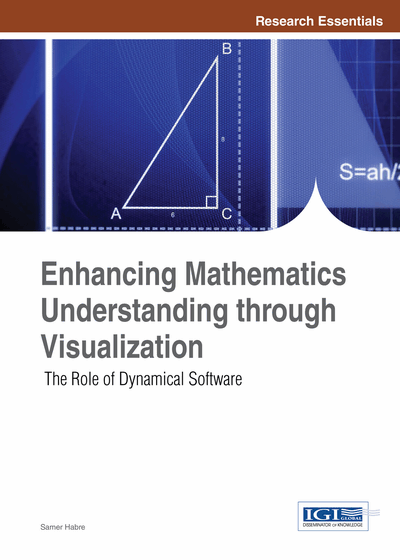# Nonlinear is Essential, Linearization is Not Enough, Visualization is Absolutely Necessary

Beverly West (Cornell University, USA)
DOI: 10.4018/978-1-4666-4050-4.ch005

## Abstract

Linear differential equations have been well understood for some time and are an important tool for studying the nonlinear systems that most frequently arise in mathematical models of real world systems. Nonlinear systems do not usually have formula solutions, but with graphics, we can see the behaviors of the solutions and thereby “understand” the differential equations. Dynamic and interactive presentations provide students with a streamlined route to understanding these behaviors, resulting in immense power and efficiency that was not previously available at the undergraduate level.
Chapter Preview
Top

## Background

### Differential Equations and their Graphs

We shall concentrate in this chapter on two-dimensional systems of autonomous differential equations, of the form. (1)

This system defines a vector field, where at any point in the xy-plane the DE tells the direction the trajectorymust follow through that point. Furthermore, there are existence and uniqueness theorems1 that guarantee, for “reasonable” functions f and g, that only one solution goes through each point in xy-space.

The xy graph of trajectories is called the phase portrait. The individual solution graphs x(t) and y(t) can also be graphed, separately, as time series or solution graphs.

## Complete Chapter List

Search this Book:
Reset